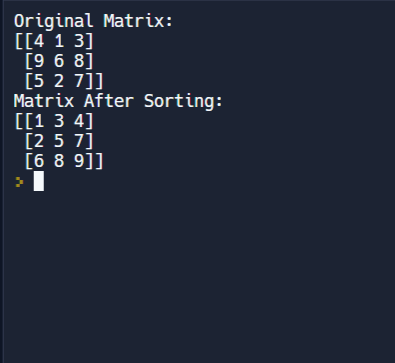# Python Program to Sort the matrix row-wise and column-wise

Given a n x n matrix. The problem is to sort the matrix row-wise and column wise.
Examples:

```Input : mat[][] = { {4, 1, 3},
{9, 6, 8},
{5, 2, 7} }
Output : 1 3 4
2 5 7
6 8 9

Input : mat[][] = { {12, 7, 1, 8},
{20, 9, 11, 2},
{15, 4, 5, 13},
{3, 18, 10, 6} }
Output : 1 5 8 12
2 6 10 15
3 7 11 18
4 9 13 20```

Approach: Following are the steps:

1. Sort each row of the matrix.
2. Get transpose of the matrix.
3. Again sort each row of the matrix.
4. Again get transpose of the matrix.

Algorithm for getting transpose of the matrix:

```for (int i = 0; i < n; i++) {
for (int j = i + 1; i < n; i++) {
int temp = mat[i][j];
mat[i][j] = mat[j][i];
mat[j][i] = temp;
}
}```

## Python 3

 `# Python 3 implementation to` `# sort the matrix row-wise` `# and column-wise` `MAX_SIZE ``=` `10`   `# function to sort each` `# row of the matrix` `def` `sortByRow(mat, n):` `    ``for` `i ``in` `range` `(n):` `        `  `        ``# sorting row number 'i'` `        ``for` `j ``in` `range``(n``-``1``):` `            ``if` `mat[i][j] > mat[i][j ``+` `1``]:` `                ``temp ``=` `mat[i][j]` `                ``mat[i][j] ``=` `mat[i][j ``+` `1``]` `                ``mat[i][j ``+` `1``] ``=` `temp`   `# function to find` `# transpose of the matrix` `def` `transpose(mat, n):` `    ``for` `i ``in` `range` `(n):` `        ``for` `j ``in` `range``(i ``+` `1``, n):`   `            ``# swapping element at` `            ``# index (i, j) by element` `            ``# at index (j, i)` `            ``t ``=` `mat[i][j]` `            ``mat[i][j] ``=` `mat[j][i]` `            ``mat[j][i] ``=` `t`   `# function to sort` `# the matrix row-wise` `# and column-wise` `def` `sortMatRowAndColWise(mat, n):` `    `  `    ``# sort rows of mat[][]` `    ``sortByRow(mat, n)`   `    ``# get transpose of mat[][]` `    ``transpose(mat, n)`   `    ``# again sort rows of mat[][]` `    ``sortByRow(mat, n)`   `    ``# again get transpose of mat[][]` `    ``transpose(mat, n)`   `# function to print the matrix` `def` `printMat(mat, n):` `    ``for` `i ``in` `range``(n):` `        ``for` `j ``in` `range``(n):` `            ``print``(``str``(mat[i][j] ), end ``=` `" "``)` `        ``print``();` `        `  `# Driver Code` `mat ``=` `[[ ``4``, ``1``, ``3` `],` `    ``[ ``9``, ``6``, ``8` `],` `    ``[ ``5``, ``2``, ``7` `]]` `n ``=` `3`   `print``(``"Original Matrix:"``)` `printMat(mat, n)`   `sortMatRowAndColWise(mat, n)`   `print``("` `Matrix After Sorting:")` `printMat(mat, n)`   `# This code is contributed` `# by ChitraNayal`

Output:

```Original Matrix:
4 1 3
9 6 8
5 2 7

Matrix After Sorting:
1 3 4
2 5 7
6 8 9```

Time Complexity: O(n2log2n).
Auxiliary Space: O(1). Please refer complete article on Sort the matrix row-wise and column-wise for more details!

Approach 2: Using NumPy

The NumPy code uses the numpy library to sort the matrix rows and transpose the matrix. The NumPy method looks considerably simple.

• np.sort() function is used to sort each row of the matrix and mat.transpose() is used to find the transpose of the matrix.
• The sortMatRowAndColWise function first sorts each row of the matrix using sortByRow function, then finds the transpose of the matrix and again sorts each row of the matrix and finds the transpose.
• The printMat function is used to print the matrix.

Note: Install numpy in python using the following command: pip install numpy

Below is the code for the above approach:

## Python3

 `import` `numpy as np`   `# function to sort each row of the matrix` `def` `sortByRow(mat):` `    ``return` `np.sort(mat, axis``=``1``)`   `# function to sort the matrix row-wise and column-wise` `def` `sortMatRowAndColWise(mat):` `    ``# sort rows of mat[][]` `    ``mat ``=` `sortByRow(mat)`   `    ``# get transpose of mat[][]` `    ``mat ``=` `mat.transpose()`   `    ``# again sort rows of mat[][]` `    ``mat ``=` `sortByRow(mat)`   `    ``# again get transpose of mat[][]` `    ``mat ``=` `mat.transpose()`   `    ``return` `mat`   `# function to print the matrix` `def` `printMat(mat):` `    ``print``(mat)`   `# Driver Code` `mat ``=` `np.array([[ ``4``, ``1``, ``3` `],` `                ``[ ``9``, ``6``, ``8` `],` `                ``[ ``5``, ``2``, ``7` `]])`   `print``(``"Original Matrix:"``)` `printMat(mat)`   `mat ``=` `sortMatRowAndColWise(mat)`   `print``(``"Matrix After Sorting:"``)` `printMat(mat)`   `# This code is contributed by adityasha4x71`

Output:Output

Time Complexity: O(n^2 log n), where n is the number of elements in the matrix.

Auxiliary Space: O(n^2), as it creates a copy of the transposed matrix.

Feeling lost in the world of random DSA topics, wasting time without progress? It's time for a change! Join our DSA course, where we'll guide you on an exciting journey to master DSA efficiently and on schedule.
Ready to dive in? Explore our Free Demo Content and join our DSA course, trusted by over 100,000 geeks!

Previous
Next